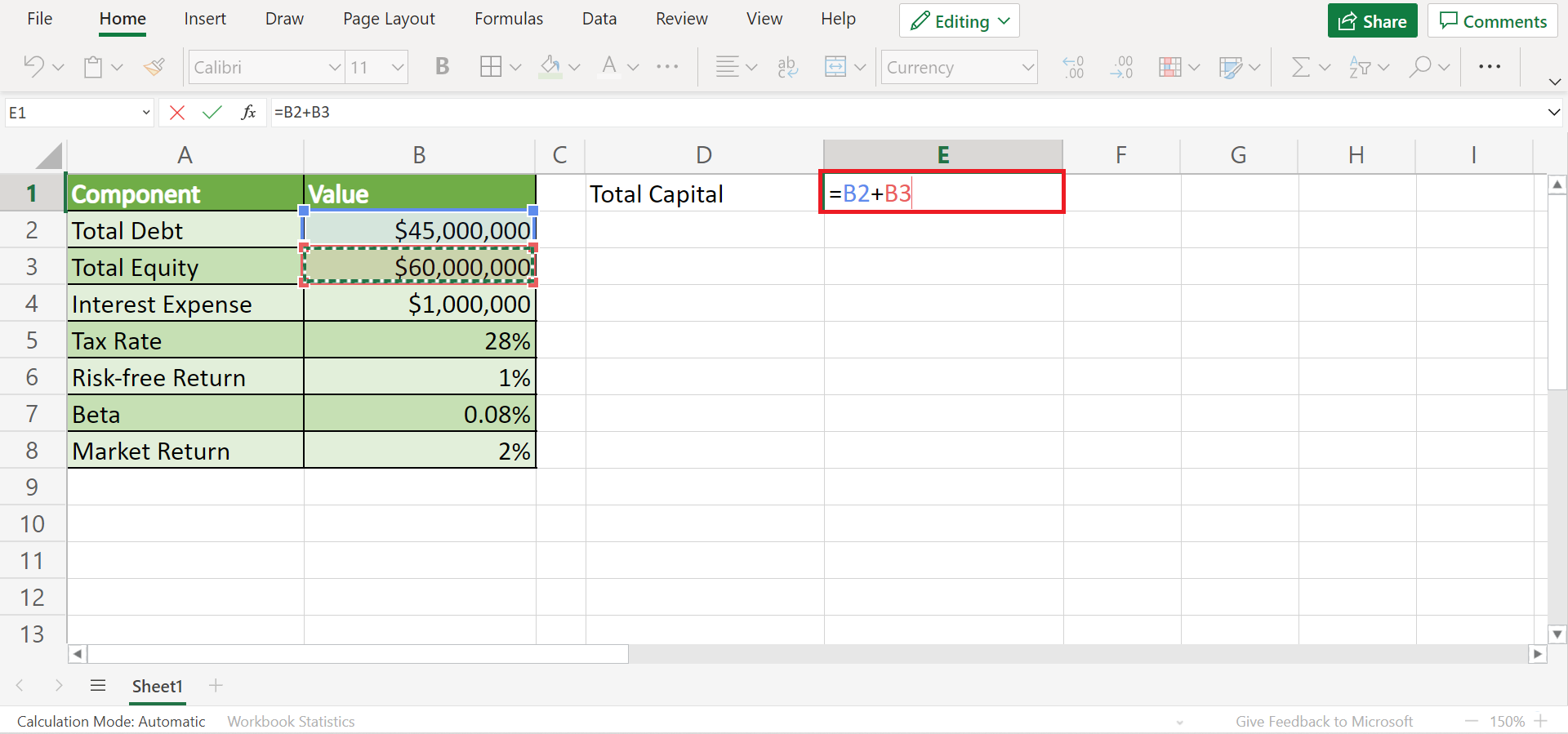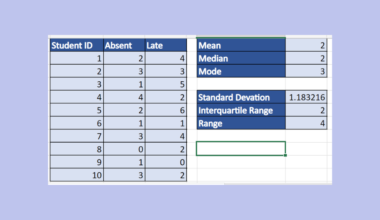# How to Calculate WACC in Excel

This guide will explain how to calculate WACC in Excel using basic arithmetic.

In other words, we need to use basic arithmetic formulas to calculate WACC successfully in Excel.

WACC stands for a weighted average cost of capital which is most commonly used in the finance field. It is also known as a simple cost of capital. Essentially, WACC calculates the rate of return a company needs to pay its debt and stakeholders.

When a company has just started, it usually borrows money or capital to fund itself. Thus, WACC calculates how much a company needs to earn to pay its debts. And it calculates this by adding the cost of debt and cost of equity together.

To calculate WACC, there are four components needed. If these components are not present, it would be impossible to calculate WACC. First is the market valuation of Equity. This refers to the total price of shares in the company.

Second, the cost of debt is the amount the company has to pay for the borrowed debt. The third is the market valuation of debt. Lastly, the cost of equity is the rate of return of shares given by the company to its shareholder.

WACC is very important, especially in the finance of a company. It is used to assess the cost of funds of a company’s capital structure. Also, investors will base their investment decisions on the WACC of a certain company.

Let’s take an example.

Suppose you are an investor. And you want to identify if a company is worth investing in or not. To make a decision, you calculate the WACC of the company. Since the process of manually calculating WACC is difficult, you used Excel to make the process easier.

If the WACC is big, you might decide not to invest in the company. If it’s low, you will take a chance and invest.

Great! Let’s move on and take a real example of calculating WACC in Excel to understand the process better.

## A Real Example of Calculating WACC in Excel

Let’s say we want to calculate a company’s WACC for a financial report.

For instance, company A is a cosmetic company with an outstanding debt of \$45 million. The company’s common equity value is \$60 million. And the company’s interest expense on its debt is \$1 million.

On the other hand, it has a 1% risk-free rate of return, a 2% market return, and a 0.08% beta. The company’s investment return is 4.5% and has a tax rate of 28%.

First, we need to prepare the necessary components to be able to calculate WACC. We can prepare a table to input all the values, which makes it easier to reference the cell and calculate in Excel.Next, we have to calculate the total capital, which is `=total debt+total equity`. From there, we calculate the weightage of debt which is =total debt/total capital. Then, the cost of debt is calculated by `=interest rate/total debt`

So weightage of equity is calculated using the formula `=total equity/total capital`. Lastly, we calculate the cost of equity which is `=risk-free return + beta*(market return-risk-free return)`.

With all these calculations, we can now calculator WACC using the formula `= weightage of equity*cost of equity + weightage of debt*cost of debt*(1-tax rate).`

Finally, the WACC of company A, a cosmetic company, is 1.26%, which is lower than the investment return of 4.5%. Thus, it is a good idea to invest in company A.Awesome! Now let’s learn the steps of calculating WACC in Excel.

## How to Calculate WACC in Excel

This section will explain the step-by-step process of calculating WACC in Excel.

1. First, create a table for all the values or data we need for calculating the components of WACC. These values are the total debt, total equity, interest expense, tax rate, risk-free return, beta, and market return.2. Second, let’s calculate total capital. Select any blank cell near the table to input the solution. In this case, let’s use cell E1. The formula is `=total debt + total equity`. Based on the cell reference, our formula is `=B2+B3`.3. Third, calculate the weightage of debt using the formula `=total debt/total capital`. In this case, the formula is =B2/E1. Input the answer in E2 to group all the components of WACC together.4. Next, let’s calculate the cost of debt using the formula `=interest expense/total debt`. So input “=B4/B2” in cell E3.5. To get the weightage of equity, input the formula “=B3/E1“ which is `=total equity/total capital`.6. Then, calculate the cost of equity, which is `=risk-free return + beta*(market return - risk-free return)`. In this case, the formula will be “=B6+B7*(B8-B6)”.7. Lastly, we can calculate WACC using all the solutions above. The formula of WACC is `=weightage of equity*cost of equity + weightage of debt*cost of debt*(1-tax rate)`.

Based on the cell reference making it easier, we will input the formula “=E4*E5+E2*E3*(1-B5)”. Press the Enter key to return the result.8. To change the WACC to a percentage value, select the cell containing the WACC and simply go to the Home tab. Then, click the dropdown menu of the Number Format. And select Percentage.9. And tada! We have calculated WACC in Excel using basic arithmetic.That’s pretty much it! Now you can successfully calculate WACC in Excel. The process may be quite long but using Excel makes everything easier. Since you only need to input the values and use cell references, you can save time and avoid errors.

Are you interested in learning more about what Excel can do? You can now use the various other Microsoft Excel formulas available to create great worksheets that work for you. Make sure to subscribe to our newsletter to be the first to know about the latest guides and tutorials from us.Our goal this year is to create lots of rich, bite-sized tutorials for Google Sheets users like you. If you liked this one, you'll love what we are working on! Readers receive ✨ early access ✨ to new content.

##### You May Also Like## How To Perform Data Binning in Excel

This guide will discuss how to perform data binning using three simple methods in Excel. Excel is a popular…## How to Extract Last Name in Excel

This guide will explain multiple methods that you can use to extract last names in Excel. We will…## How to Use KURT Function in Excel

This guide will explain how to use the KURT function in Excel. When performing statistical analysis on a…## How to Lookup a Value in a 3D Table in Excel

This guide will explain how you can use Excel functions to lookup values in a 3D table in…